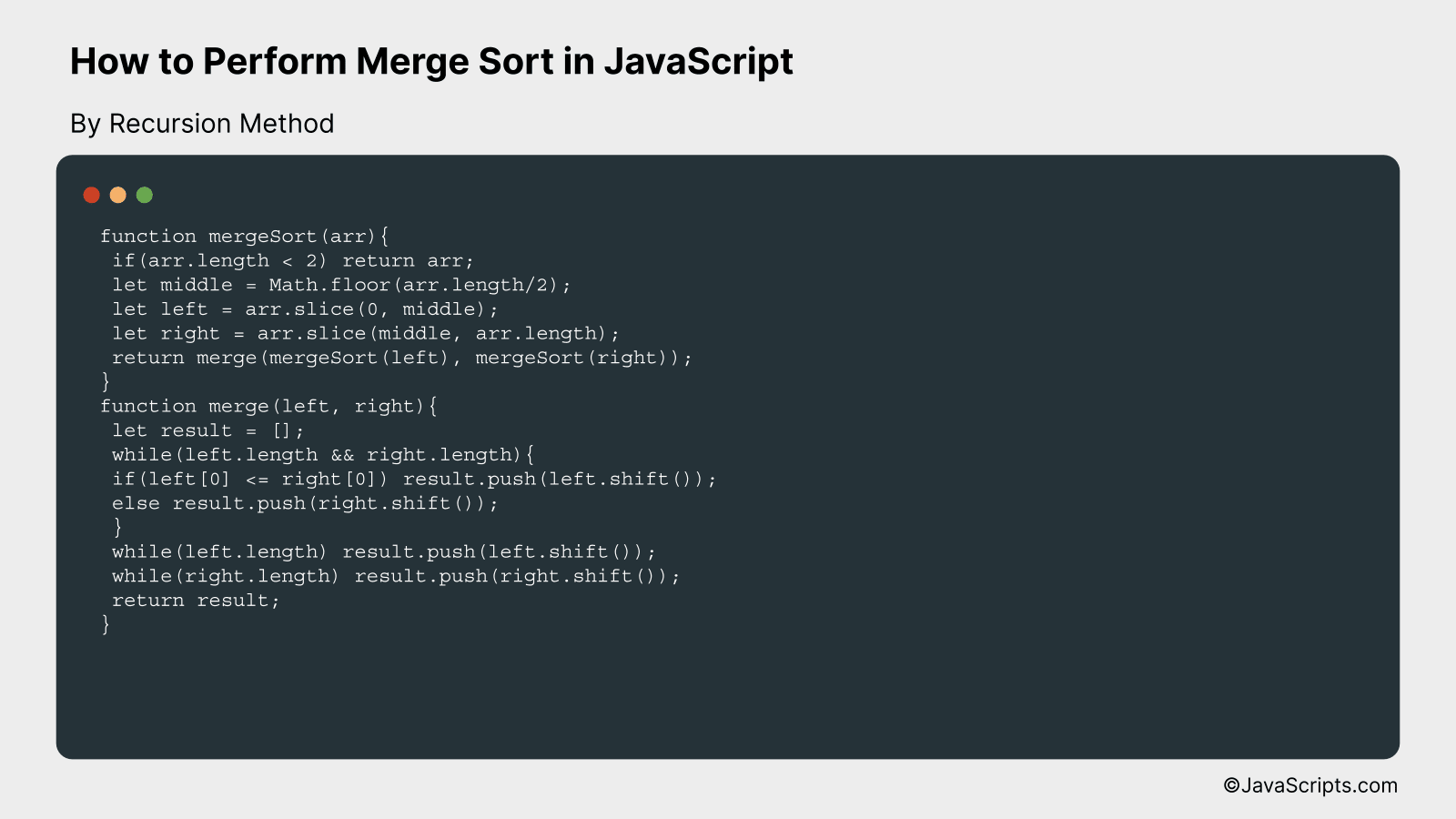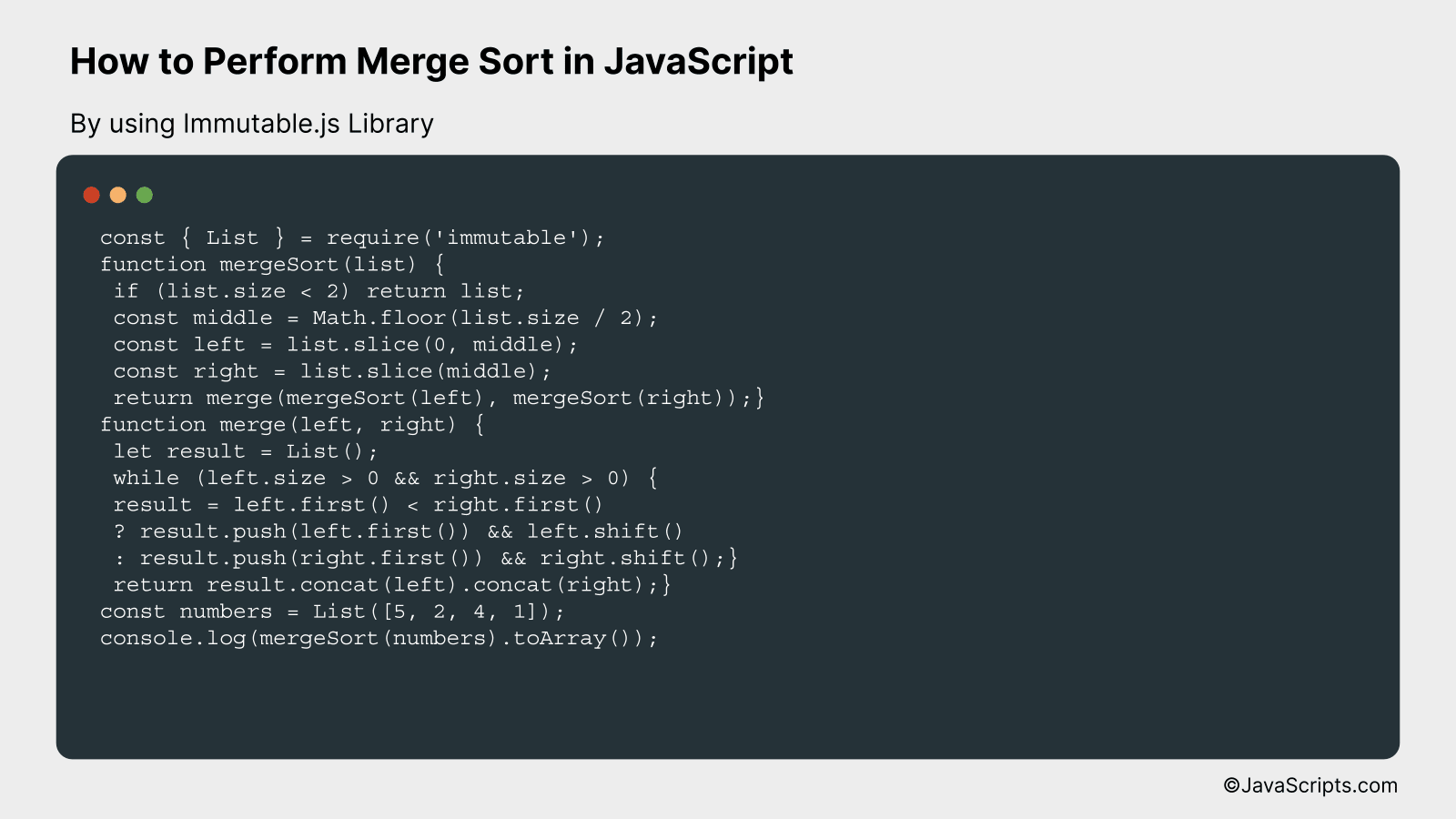# How to Perform Merge Sort in JavaScript

Let’s dive into the world of JavaScript and explore one of its most fundamental algorithms – the Merge Sort. Often found in the toolbox of developers, this powerful sorting algorithm comes in handy when dealing with extensive lists and datasets.

You and I will break down the Merge Sort in manageable steps, making it easy to understand, even for beginners. With the aid of examples and simple explanations, we will unravel the logic behind this algorithm and how it performs to sort data efficiently.

In this post, we will be looking at the following 3 ways to perform merge sort in JavaScript:

• By Recursion Method
• By using the JavaScript Spread Operator
• By using Immutable.js Library

Let’s explore each…

## #1 – By Recursion MethodMerge sort is a divide-and-conquer algorithm that continuously splits a list in half. If the list is empty or has one item, it is sorted by definition. The list is then recursively split into two halves until it can no longer be split. Once the splitting is done, the merging process begins. As an example, if you have the list [38, 27, 43, 3, 9, 82, 10], the algorithm will split this into [38, 27, 43] and [3, 9, 82, 10], sort them, and merge them back together in the correct order.

``````
function mergeSort(arr){
if(arr.length < 2) return arr;
let middle = Math.floor(arr.length/2);
let left = arr.slice(0, middle);
let right = arr.slice(middle, arr.length);
return merge(mergeSort(left), mergeSort(right));
}
function merge(left, right){
let result = [];
while(left.length && right.length){
if(left <= right) result.push(left.shift());
else result.push(right.shift());
}
while(left.length) result.push(left.shift());
while(right.length) result.push(right.shift());
return result;
}
``````

### How it Works

Merge sort works by dividing the unsorted list into n sublists, each containing one element (a list of one element is considered sorted), and repeatedly merging sublists to produce new sorted sublists until there is only one sublist remaining. This results in the list being sorted.

• It starts by checking if the array length is less than 2, in which case it just returns the array since it's already sorted.
• Then, it finds the middle of the array and splits the array into two halves, left and right.
• It then recursively calls the mergeSort function on these halves. The recursion continues until we are dealing with arrays of length 0 or 1.
• Once the array has been split enough times, it starts merging them back together with the merge function. This function continuously compares the first elements of the left and right halves and pushes the smaller one into the result array.
• The merge function continues this process until both the left and right arrays are empty, ensuring that all elements have been sorted and added to the result array.
• The sorted array is then returned.

## #2 - By using the JavaScript Spread OperatorMerge sort in JavaScript using the spread operator works by dividing the array into two halves, recursively sorting each half, and then merging them back together in sorted order. For instance, you can imagine the array [4, 3, 2, 1] being split into [4, 3] and [2, 1], sorted separately, then merged back to form the sorted array [1, 2, 3, 4].

``````
function mergeSort(arr) {
if (arr.length <= 1) {
return arr;}
const mid = Math.floor(arr.length / 2);
const left = arr.slice(0, mid);
const right = arr.slice(mid);
return merge(
mergeSort(left),
mergeSort(right));}
function merge(left, right) {
let result = [];
while (left.length && right.length) {
if (left <= right) {
result.push(left.shift());
} else {
result.push(right.shift());}}
return [...result, ...left, ...right];}
``````

### How it works

This implementation of merge sort works by continually dividing the input array into two halves until we are dealing with arrays that only contain one element (or no elements), which are naturally sorted. Then, these smaller arrays are merged back together in sorted order.

• The function `mergeSort` takes an array as input. If the array has one or no elements, it returns the array itself, as there is nothing to sort.
• We calculate the middle index of the array, and use the JavaScript `slice` function to split the array into two halves: 'left' and 'right'.
• We then recursively call `mergeSort` on the left and right halves. This continues until we reach arrays that are small enough to be naturally sorted (arrays of size 0 or 1).
• The function `merge` is used to merge two sorted arrays (left and right) together. It creates an empty result array, and compares the first elements of the left and right arrays, pushing the smaller one into the result array.
• If one of the arrays runs out of elements, we use the JavaScript spread operator (`...`) to append all remaining elements from the other array to the result.
• The sorted array is then returned. `mergeSort` continues to merge sorted arrays together, working its way up until the entire array is sorted.

## #3 - By using Immutable.js LibraryMerge Sort is a divide and conquer algorithm that splits a list into two equally-sized halves, recursively sorts them, and then merges them back together. Using Immutable.js, we'll create an immutable version of this sorting algorithm in JavaScript. Let's assume we are sorting a short list of numbers: [5, 2, 4, 1]

``````
const { List } = require('immutable');
function mergeSort(list) {
if (list.size < 2) return list;
const middle = Math.floor(list.size / 2);
const left = list.slice(0, middle);
const right = list.slice(middle);
return merge(mergeSort(left), mergeSort(right));}
function merge(left, right) {
let result = List();
while (left.size > 0 && right.size > 0) {
result = left.first() < right.first()
? result.push(left.first()) && left.shift()
: result.push(right.first()) && right.shift();}
return result.concat(left).concat(right);}
const numbers = List([5, 2, 4, 1]);
console.log(mergeSort(numbers).toArray());
``````

### How it works

The mergeSort function recursively divides the input list into two halves until it is small enough to sort directly (when the size is less than 2). After sorting each half, they are merged back together in the correct order using the merge function.

• First, the function checks if the list size is less than 2. If so, it returns the list as it is already sorted.
• The list is split into two halves at the middle index.
• The function is called recursively on both halves, which further divides the list until it has only one element.
• The merge function is then used to combine these sorted halves. It compares the first elements of the left and right lists, pushing the smaller one to the result, and removing it from its original list.
• This process continues until one of the lists is empty. Afterwards, any remaining elements from the other list are concatenated to the result.
• The sorted list is then returned.

Related:

To conclude, merge sort is a highly efficient sorting method that’s easy to implement in JavaScript. By breaking down an array into smaller pieces and merging them back together in a sorted manner, we can effectively organize data.

Remember, the key is to understand the core concept of 'divide and conquer'. With practice, implementing merge sort or any other algorithm in JavaScript (or any other language) becomes almost second nature. Keep coding and keep improving!